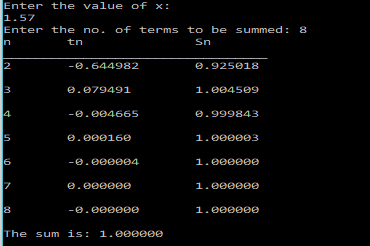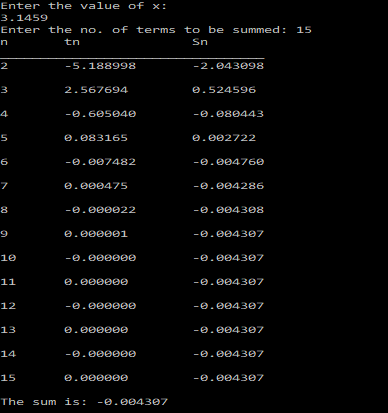# Sine Series Finite Sum – C PROGRAM

In the last post, I discussed about how would one go about calculating the sum of a finite series using C.

In this post I will apply that method, to find the sum of the Sine series for only a finite number of terms.

Sine series is given by:$\sin (x) = x-\frac{x^3}{3!} + \frac{x^5}{5!} - ....$

We will start the numbering the terms from 0. That is,$t_0=x$ ,$t_1=-\frac{x^3}{3!}$, ….

It’s easy to see that the ratio between consecutive terms is given by:$R=\frac{t_i}{t_{i-1}}=-\frac{x^2}{(2i+1)2i}$

Since, we indexed the terms starting from 0, therefore, for the above relation to work,$i$ will go from 1 to$n$.

[Hint: To find the general form of the ratio given in the above expression, try writing down t1/t0, t2/t1,…and then you would be able to see the ratio.]

Now, knowing the first($t_0$) term, the successive terms can be calculated as :$t_1=R\times t_0$$t_2=R\times t_1$

and so on.

Therfore, the C program that calculates the sum of the sin series upto a given number of terms can be written as shown below.

### PROGRAM:

/********************************
******FINITE SERIES SUM**********
Series: sin(x) = x - (x^3/3!) + (x^5/5!) + .....
********************************/
#include<stdio.h>
#include<math.h>
main(){
int i,n;
double x,t0,t1,R,sum;
printf("Enter the value of x:\n");
scanf("%lf",&x);
printf("Enter the no. of terms to be summed: ");
scanf("%d",&n);
//Initialize First Term
t0=x;
//Make sum equal to the first term
sum=t0;
printf("n\ttn\t\tSn\n_________________________________");
for(i=1;i<n;i++){
//Find the ratio of the second term to the first term using already known relation
R=-(x*x)/(2*i+1)/(2*i);
//Calculate the second term
t1=R*t0;
//find the new sum
sum=sum+t1;
t0=t1;
printf("\n%d\t%f\t%lf\n",i+1,t1,sum);
}
printf("\nThe sum is: %f",sum);
}


The program also prints the value of each term(except the first($t_0$) term) and sum(partial) upto that term.

### OUTPUT:

The output of the above program for various values of$x$ and no. of terms is shown below: